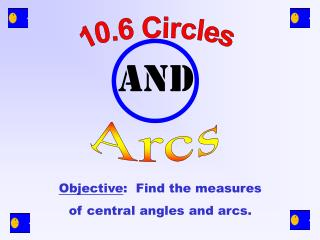DownloadDownload Presentation10.6 Circles

# 10.6 Circles

Télécharger la présentation## 10.6 Circles

- - - - - - - - - - - - - - - - - - - - - - - - - - - E N D - - - - - - - - - - - - - - - - - - - - - - - - - - -
##### Presentation Transcript

1. 10.6 Circles and Arcs Objective: Find the measures of central angles and arcs.

2. A CIRCLEis the set of all points equidistant from a given point called the center. This is circle P for Pacman. Circle P P

3. Central angle A CENTRAL ANGLEof a circle is an angle with its vertex at the center of the circle.

4. Arc An arc is a part of a circle. In this case it is the part Pacman would eat. 

5. Semicircle ABC m ABC = 180 One type of arc, a semicircle, is half of a circle. C P B A

6. A minor arc is smaller than a semicircle. A major arc is greater than a semicircle. less than 180 more than 180

7. LMN is a major arc. mLMN = 360 – mLN RS is a minor arc. mRS = m RPS. S P R N O M L

8. Identify the following in circle O: 1) the minor arcs C A O E D

9. Identify the following in circle O: 2) the semicircles C A O E D

10. Identify the following in circle O: 3) the major arcs containing point A C A O E D

11. R 53o 53o P O The measure of a central angle is equal to its intercepted arc.

12. Find the measure of each arc. • BC = 32 • BD = 90 • ABC = 180 • AB = 148

13. Here is a circle graph that shows how people really spend their time. Find the measure of each central angle in degrees. • Sleep • Food • Work • Must Do • Entertainment • Other

14. THE END Homework: page 569 (1 – 26)